Related Articles

# MATLAB Annotation

• Last Updated : 28 Apr, 2021

Annotations in MATLAB is a way of adding explanation or notes to the plots. Annotations add more information to the plots. There are different syntax formations for adding annotations to a plot:

• annotation(lineType,x,y)
• annotation(lineType)
• annotation(shapeType,dim)
• annotation(shapeType)
• annotation(___,Name,Value)

Let’s discuss all the above functions in detail:

## annotation(lineType,x,y)

• Creates a line or arrow annotation between two points in the current figure.
• lineType takes different values as ‘line’, ‘arrow’, ‘doublearrow’, or ‘textarrow’.
• x and y are two-element vectors of the form [x_begin x_end] and [y_begin y_end], respectively.
• Annotation has starting point as (x_begin,y_begin) and ending point as (x_end,y_end).

Example :

• Draw a plot y = x using plot(1:10).
• Specify x and y values i.e starting point as (0.4, 0.8) and ending point as (0.6. 0.6).
• Specify lineType as ‘arrow’ with x and y as two-element vectors.

## Matlab

 `% Plots y = x line from 1 to 10``plot(1:10)`` ` `x = [0.4 0.6];``y = [0.8 0.6];`` ` `% annotation with lineType 'arrow'``annotation(``'arrow'``,x,y)`

Output :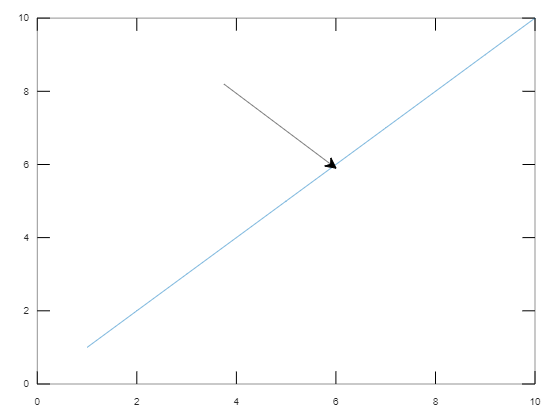## annotation(lineType)

• Creates an annotation of specified “lineType” with default position starting from (0.3, 0.3) and ending at (0.4, 0.4).

Example :

• Draw a plot y = -x.
• Specify lineType as ‘arrow’.

## Matlab

 `% Plot y = -x from 1 to 10``x = [1:10]``y = -x``plot(x,y)`` ` `% annotation of lineType 'arrow'``% at default positions``annotation(``'arrow'``)`

Output :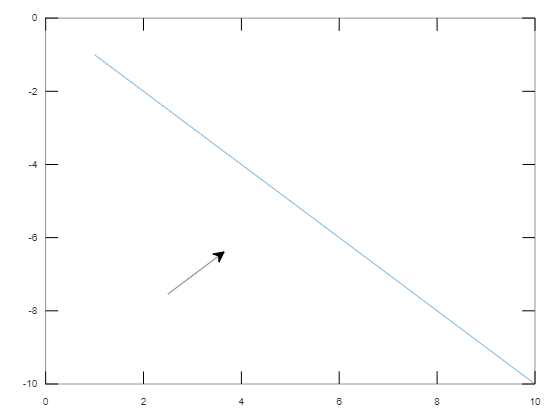## annotation(shapeType,dim)

• Creates an annotation in the shape of a rectangle or eclipse with given dim to the plot.
• ShapeType takes values as ‘rectangle’, ‘ellipse’, or ‘textbox‘.
• dim is a vector of size 4 as [x y w h], where (x, y) is the lower-left endpoint of rectangle and w, h are width and height of rectangle respectively.

Example :

• Plot the graph y=x^2 from 0 to 10.
• Specify dim as the lower-left endpoint (0.2, 0.3) with width and height as 0.3 and 0.3 respectively.
• Specify annotation of shape textbox by including a string in the textbox.

## Matlab

 `% Plot y = x^2 from 0 to 10``x = [0:10]``y = x.*x``plot(x,y)`` ` `% Dimensions of textbox``dim = [0.2 0.3 0.3 0.3]``str = ``'Parabola y = x^2'``;`` ` `% Annotation of shapeType 'textbox'``% at "dim" with "str"``% content inside the textbox``annotation(``'textbox'``,dim,``'String'``,str);`

Output :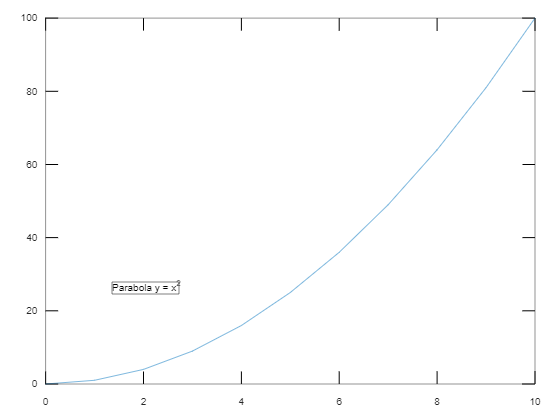## annotation(shapeType)

• Creates the annotation with the mentioned shape in the default position so that the lower-left endpoint is at (0.3, 0.3) and the width and height are both 0.1.

Example :

• Plot the graph y = x^2.
• Create an annotation of shapeType = ‘rectangle’ with default positions of the rectangle.

## Matlab

 `% Plot y = ^2 from 0 to 10``x = [0:10]``y = x.*x``plot(x,y)`` ` `% Annotation with shapeType='rectangle'``% with default positions ``% of rectangle``annotation(``'rectangle'``);`

Output :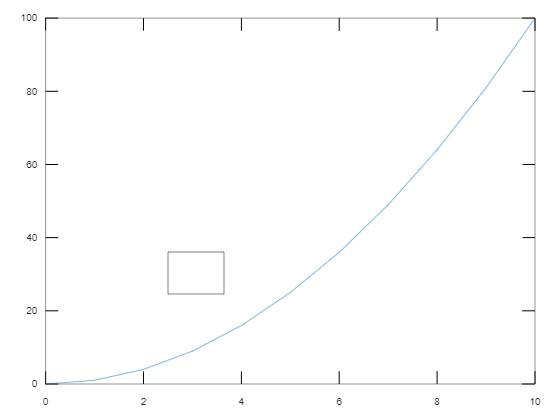## annotation(___,Name,Value)

• Creates an annotation by specifying properties as Name-Value pair arguments.
• Some of the properties are like String, color, FaceColor, FaceAlpha etc.

Example :

• Plot the graph y = x^3-12x.
• Specified the eclipse annotation with color as ‘red’ and interior color as ‘green’ and FaceAlpha = 0.3. specifies FaceColor is slightly transparent.

## Matlab

 `% Plot y = x^3 - 12x from -5 to +5``x = linspace(-5,5);``y = x.^3 - 12*x;``plot(x,y)`` ` `% Dimensions of eclipse``dim = [.3 .50 .25 .15];`` ` `% eclipse takes dimensions as it'll``% fit into specified``% rectangle dimension``% Anootation with color , FaceColoe and``% FaceALpha of eclipse``annotation(``'ellipse'``,dim,``'color'``,``'red'``,``'FaceColor'``,``'green'``,``'FaceAlpha'``,.3)`

Output :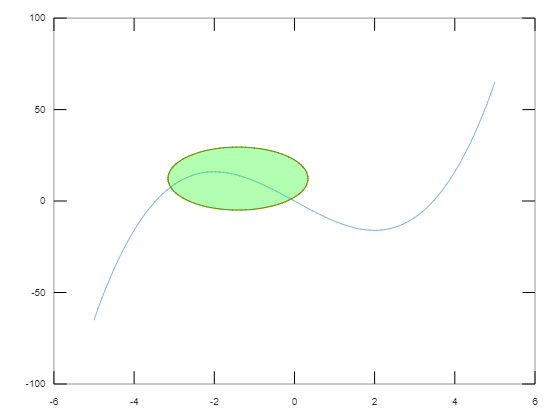My Personal Notes arrow_drop_up